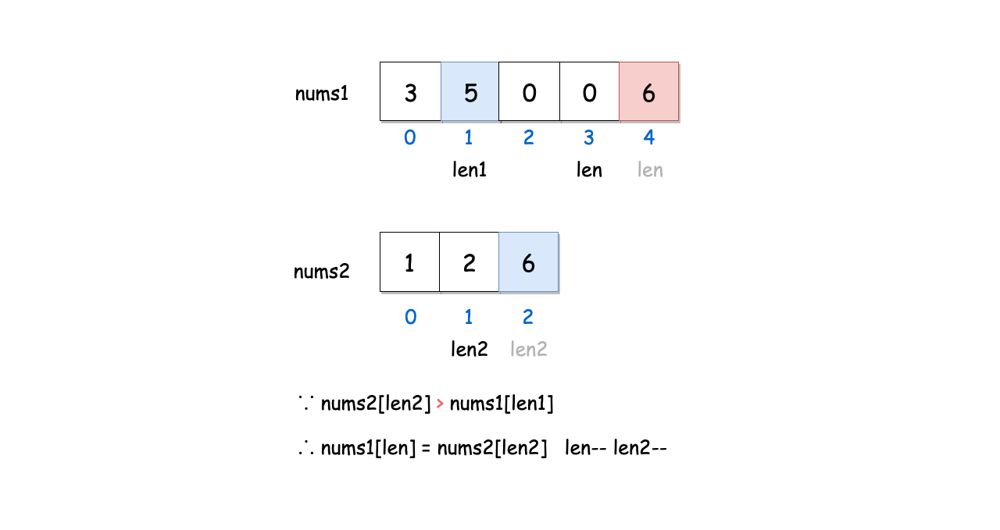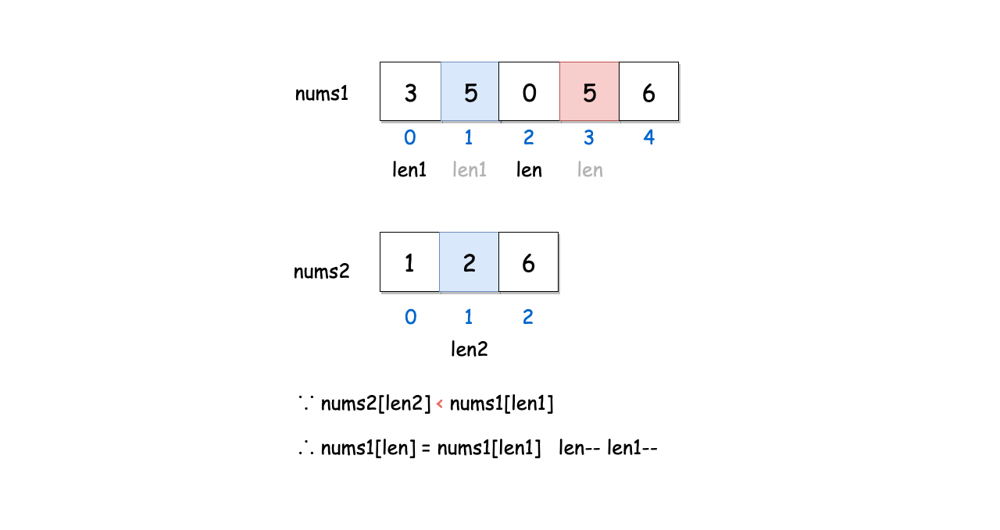By Long Luo

Here shows 3 Approaches to slove this problem: Sorting, Two Pointers and Reverse Two Pointers.

# Sorting

Let the array $\textit{nums}_2$ into the rear of array $\textit{nums}_1$, and then sort the entire array.

## Analysis

• Time Complexity: $O((m + n)log(m+n))$.
• Space Complexity: $O(log(m+n))$.

# Two Pointers

Since two arrays $\textit{nums}_1$ and $\textit{nums}_2$ are both sorted in non-decreasing order, so we can use the Two Pointers method.

We consider the two arrays as two queues, takes the smaller numbers from the two arrays headers each round and put it into our results.

## Analysis

• Time Complexity: $O(m + n)$.
• Space Complexity: $O(m + n)$.

# Reverse Two Pointers

Since the extra space of $\textit{nums}_1$ is in the back, it’s better to process the sorted data from the back to the front, fill in the values while traversing the array.

Let two pointers $len1$ and $len2$ to point to the tails of $nums1$ and $nums2$, traversal from the tail and compare the values, and set the pointer $len$ to point to the end of $\textit{nums}_1$. After each traversal of the size of the comparison value, it will be filled.

When $len1 \lt 0$, the traversal ends. If the data of $\textit{nums}_2$ is not copied completely, we can copy the rest to the front of $\textit{nums}_1$, and finally we got the answer.

The process is show as the following images.## Analysis

• Time Complexity: $O(m + n)$.
• Space Complexity: $O(1)$.

All suggestions are welcome.
If you have any query or suggestion please comment below.
Please upvote👍 if you like💗 it. Thank you:-)

Explore More Leetcode Solutions. 😉😃💗